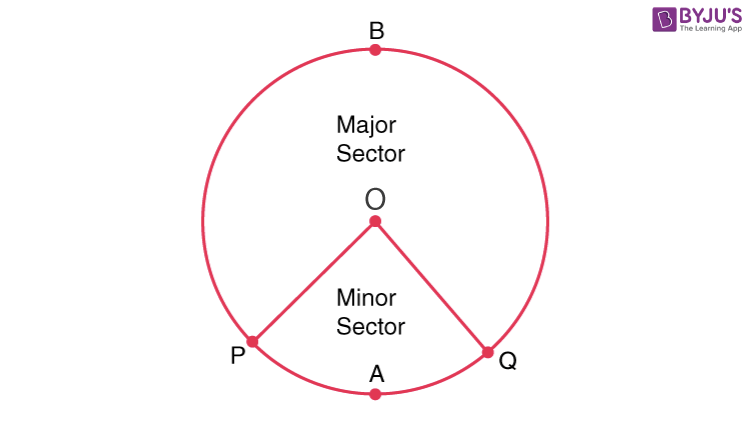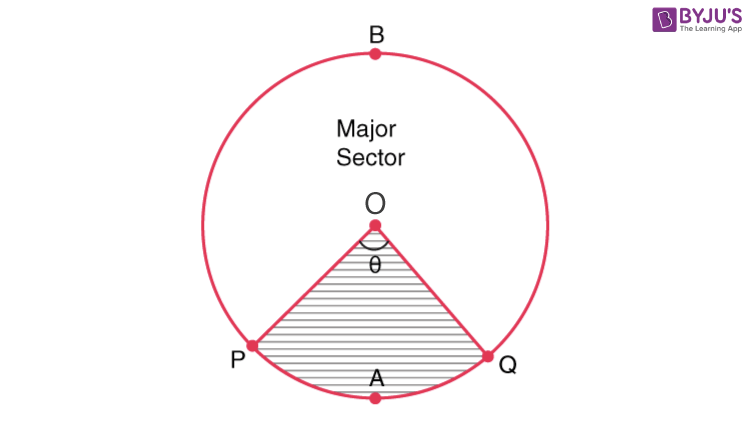# Area Of Sector Of A Circle

Before knowing about a sector of a circle, let’s know how the area of a circle is calculated. When it comes to the area, it is always related to two dimensions. Anything which is two-dimensional can form a plane. So, any two-dimensional figure will have an area. What about a circle?

Definition 1: A circle is the collection of all the points in a plane which are at a fixed distance from a fixed point. The fixed point is known as the center of the circle and the fixed distance is known as the radius of the circle.

Definition 2: If all the points which lie inside and on the circle are taken together, the plane constructed is known as a disk.

A disk is basically the region bounded by a circle. So, the area of a circle will always be that of the disk. The area, A of the circle with radius r is given by

$$\begin{array}{l}A = \pi r^{2}\end{array}$$

Definition 3: The portion of the circle enclosed by two radii and the corresponding arc is known as the sector of a circle.

Basically, a sector is the portion of a circle. It would hence be right to say that a semi-circle or a quarter-circle is a sector of the given circle. In fig.1, OPAQ is called the minor sector and OPBQ is called the major sector because of lesser and greater areas. The angles subtended by the arcs PAQ and PBQ are equal to the angle of the sectors OPAQ and OPBQ respectively. When the angle of the sector is equal to 180°, there is no minor or major sector.## Area of sector

In a circle with radius r and center at O, let ∠POQ = θ (in degrees) be the angle of the sector. Then, the area of the circle is calculated using the unitary method.When the angle of the sector is 360° (i.e., the whole circle), Then the area of the sector is:

$$\begin{array}{l}A = \pi r^{2}\end{array}$$

When the angle is 1°, then the area of a sector is:

$$\begin{array}{l}A = \frac{\pi r^{2}}{360^{\circ}}\end{array}$$

So, when the angle is θ, area of sector, OPAQ,

$$\begin{array}{l}A = \frac{\theta}{360^{\circ}}~\times ~ \pi r^{2}\end{array}$$

Similarly, length of the arc (PQ) of the sector with angle θ,

$$\begin{array}{l}l = \frac{\theta }{360^{\circ}}\times 2\pi r\end{array}$$

If the length of the arc of the sector is given instead of the angle of the sector, there is a different way to calculate the area of the sector. Let the length of the arc be l. For the radius of a circle equal to r units, an arc of length r units will subtend 1 radian at the center. It can be hence concluded that an arc of length l will subtend l/r angle at the center. So, if l is the length of the arc, r is the radius of circle and θ is the angle subtended at center, θ = l/r.

When angle of the sector is 2π, area of the sector i.e. the whole circle is

$$\begin{array}{l}A = \pi r^{2}\end{array}$$

When the angle is 1, the area of the sector is:

$$\begin{array}{l}A = \frac{\pi r^{2}}{2\pi } = \frac{r^{2}}{2}\end{array}$$

So, when the angle is θ, area of the sector is:

$$\begin{array}{l}A = \theta ~\times ~\frac{r^{2}}{2}\end{array}$$
$$\begin{array}{l}A = \frac{l}{r}~\times ~\frac{r^{2}}{2}\end{array}$$
$$\begin{array}{l}A = \frac{lr}{2}\end{array}$$

Some examples for better understanding are discussed from here on.

Example 1:

If the angle of the sector with a radius of 4 units is 45° then find the area and length of the sector.

Solution:

We know that,

$$\begin{array}{l}A = \frac{\theta }{360^{\circ}}~\times ~ \pi r^{2}\end{array}$$
$$\begin{array}{l}A = \frac{45^{\circ}}{360^{\circ}}~\times ~\frac{22}{7}~\times ~4~\times ~4\end{array}$$
$$\begin{array}{l}A = \frac{44}{7}square\ units\end{array}$$

The length of the same sector is

$$\begin{array}{l}l = \frac{\theta }{360^{\circ}}~\times ~ 2\pi r\end{array}$$
$$\begin{array}{l}l = \frac{45^{\circ}}{360^{\circ}}~\times ~2~\times ~\frac{22}{7}~\times ~4\end{array}$$
$$\begin{array}{l}l = \frac{22}{7}\ units\end{array}$$

Example 2:

If the length of the arc of a circle with a radius of 16 units is 5 units, then find the area of the sector corresponding to that arc?

Solution:

Given: l = 5 units, r = 16 units

As we know, A = (lr)/2

Now, substitute the values in the formula, we get

A = [16 × 5]/2

A = 80/2

A = 40

Therefore, the area of the sector corresponding to the arc is 40 square units.

To practice more on are of the sector of a circle, download BYJU’S – The Learning App from the Google Play Store.

## Frequently Asked Questions on Area of Sector of a Circle

Q1

### What is the formula for the area of a sector of a circle?

The formula for the area of the sector of a circle is (θ/360°) × πr2.
Where r is the radius of the circle and 𝜃 is the angle of the sector.

Q2

### What is the length of the arc of the sector with angle θ?

The length of the arc of the sector, l is (θ/360°) × 2πr

Q3

### When the angle is 1, what is the area of the sector?

When the angle is 1, then the area of the sector is r2/2

Q4

### If the length of the arc of a circle with a radius is given, what is the area of the sector corresponding to that arc?

When the angle is θ, the area of the sector is lr/2.

Test your knowledge on Area Of Sector